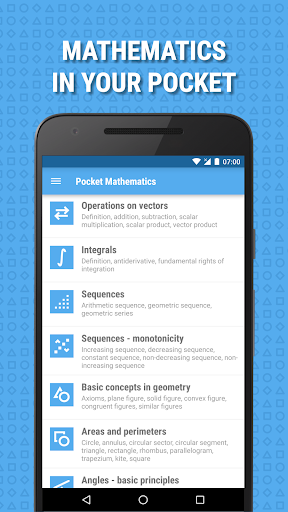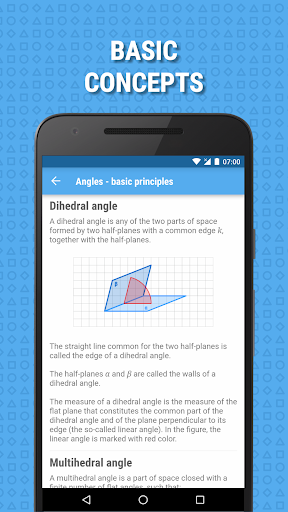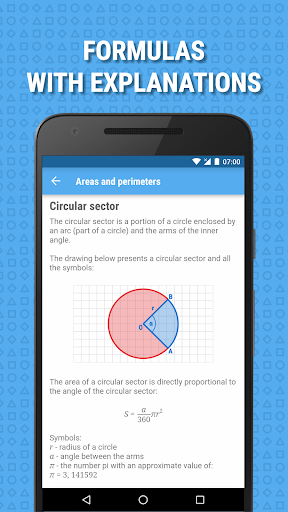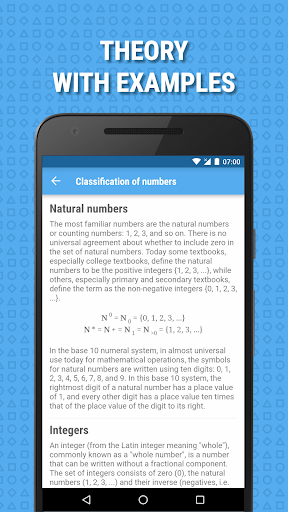# Pocket Mathematics APK

## gecko.droid.mathematicshelperPocket Mathematics is easy to use, free education app that covers most of the vital concepts, equations, and formulas of mathematics. This education application is a must-have guide, whether you want to refresh your knowledge, prepare for an exam, or just refresh the core concepts of mathematics. Pocket Mathematics provides discrete explanations of critical concepts taught in an introductory math course from logic and sets to integrals. It is also a perfect reference, full of formulas, equations, and images for students who need help with math homework assignments.

Key features:
– Contains content focused on key topics only
– Each topic contains formulas, equations and detailed description with images
– Perfect for students to do math homework quickly and accurately
– Great for review critical mathematics concepts
– Suitable for all levels of mathematics from primary school to university

Pocket Mathematics is the best math app on Android market. All this features in this app and will make your mathematical life a lot easier. This educational app provides free math lessons and math homework help from basic math to complex problems.

This math application contains the following topics:
– Mathematical logic
– Sets
– Classification of numbers
– Expressions and actions
– Exponentiation
– Average values
– Functions
– Monotonicity of function
– Derivative of a function
– Operations on vectors
– Integrals
– Sequences
– Areas and perimeters

Supported languages:
– English
– Polish
– Spanish (beta)

Website: http://www.geckonization.com

#### What’s New

• bug fixes and performance improvements